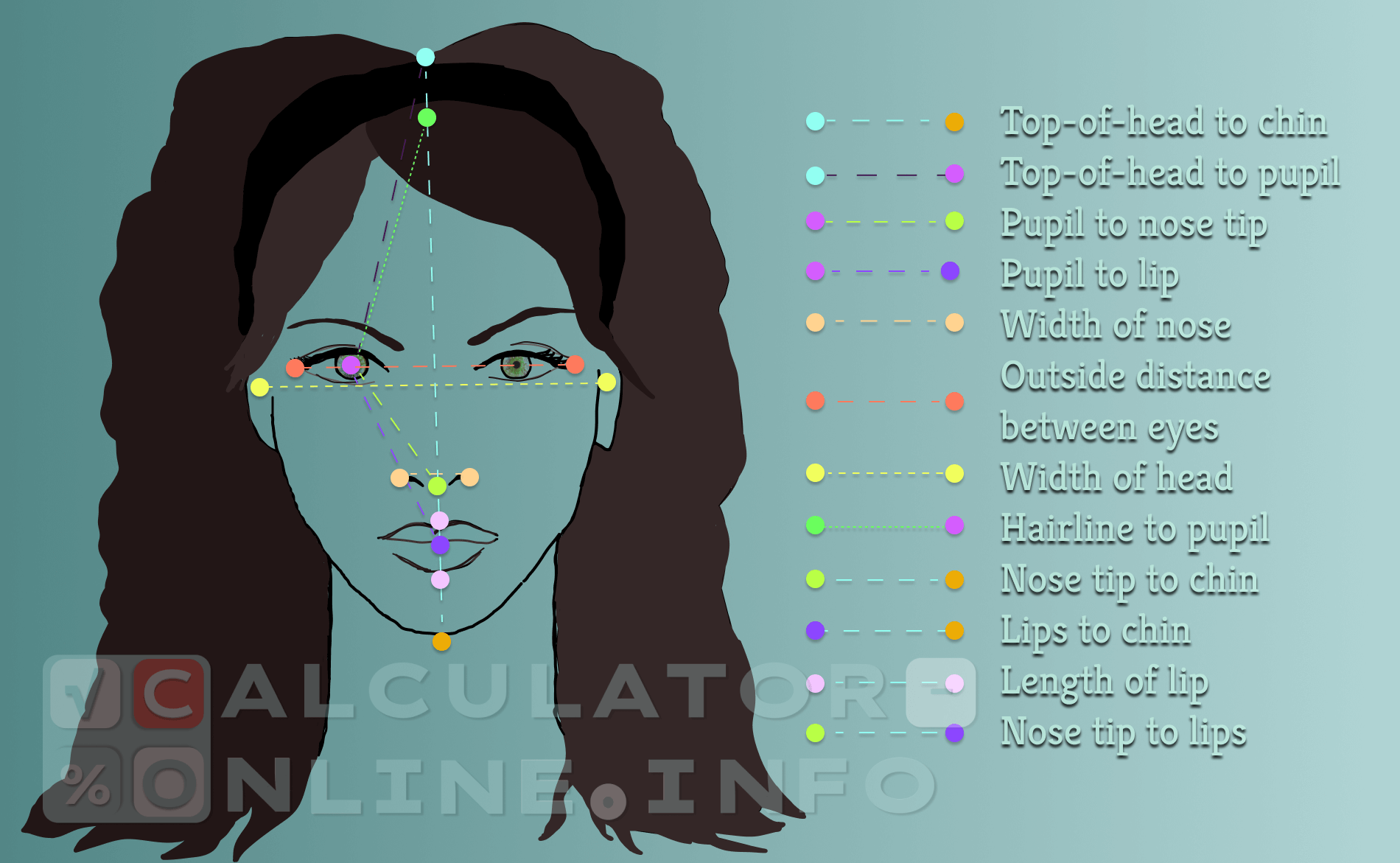# Calculate the golden ratio face | Know if your proportions are ideal

Golden ratio face calculator is the tool that helps to determine if facial parameters correspond to the rule of the golden beauty ratio.

## What is the golden ratio of face?

According to the golden ratio the distance between certain areas of your face to the distance of other defined areas must be as close to 1.618 as it possible. The reason is that some researchers have discovered the relation between human perception of physical beauty and the golden ratio. That’s why the closer the obtained indicators, the more beautiful the person’s face is considered.## Golden ratio face test

For the calculation you need to know such golden ratio face measurements as these distances:

3. Pupil to nose tip;
4. Pupil to lip;
5. Width of nose;
6. Outside distance between eyes;
8. Hairline to pupil;
9. Nose tip to chin;
10. Lips to chin;
11. Length of lips;
12. Nose tip to lips.

To calculate golden ratio, face proportions should be measured directly from a person.

## How to use golden ratio face calculator?

This is a general calculator for women and men, so gender is not required. You can use it as a female golden ratio face calculator as well as male golden ratio face calculator.

When the measurements are ready you can calculate golden ratio face score. You should enter all numbers in centimeters in the gabs and put on the button “Calculate”.

Rating
( 11 assessment, average 4.45 from 5 )Mathematician/ author of the article